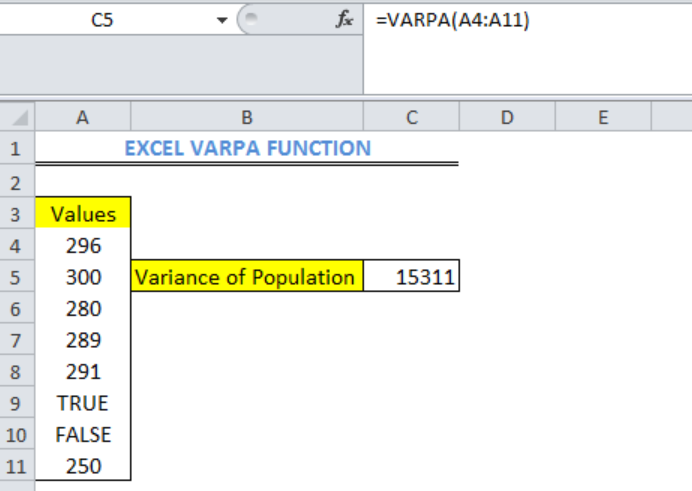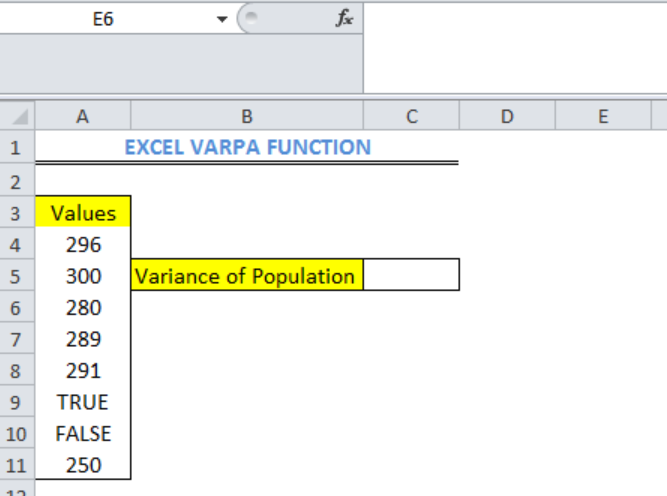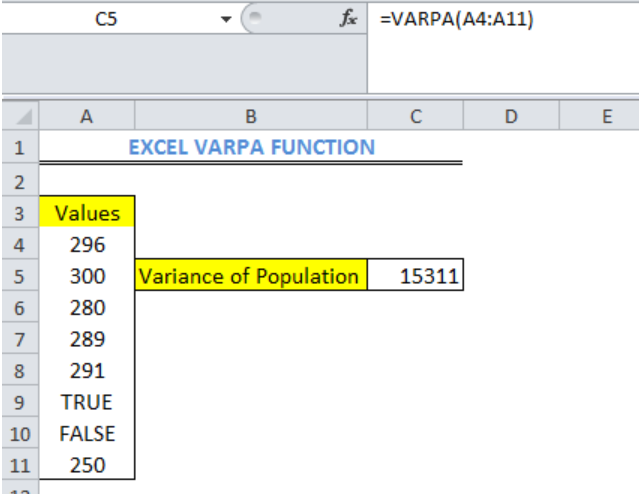Get instant live expert help with Excel or Google Sheets“My Excelchat expert helped me in less than 20 minutes, saving me what would have been 5 hours of work!”

#### Post your problem and you’ll get Expert help in seconds.

Your message must be at least 40 characters
Our professional Expert are available now. Your privacy is guaranteed.

# Learn How to Use the Excel VARPA Function

We can use the statistical Excel VARPA function to calculate the variance of a population. This calculation can include text and logical values. Logical values like TRUE, FALSE and TEXT has the values of 1, 0 and 0 respectively. This article will step through the use of the VARPA function.Figure 1: Variance of Population with the Excel VARPA Function

## The syntax for Excel VARPA function

`=VARPA(Value1, [Value2, ... Value_n])`

## Formula

`=VARPA(A4:A11)`

## Setting up the Data

We will set up the data according to the content of figure 2.

• The values whose variance we intend to calculate are in Column A.
• The output or result of the variance of a population will be in Cell C5Figure 2: How to Use the Excel VARPA Function

## Using the VARPA Function

• We will click on Cell C5 and insert the formula: `=VARPA(A4:A11)`

• We will press the enter keyFigure 3: Variance of Population with the Excel VARPA Function

## Note

1. The EXCEL VARPA Function is used for arguments that represent only an entire population. For values that represent a sample of a population, the Excel VARA function is used.
2. We can ignore logical and text values in references with the Excel VARP or VAR.P function to calculate variance based on an entire population.
3. Empty cells in arrays or cell references are ignored in EXCEL VARPA Function

## Instant Connection to an Expert through our Excelchat Service

Most of the time, the problem you will need to solve will be more complex than a simple application of a formula or function. If you want to save hours of research and frustration, try our live Excelchat service! Our Excel Experts are available 24/7 to answer any Excel question you may have. We guarantee a connection within 30 seconds and a customized solution within 20 minutes.

Solution examplesUse the Vlookup Function to complete the "employee" column of table 2. Use "job Id" from table 2 as your lookup_value(s) and table 1 as your reference.
Solved by C. H. in 16 minsIf a cell in another sheet is populated I need a vlookup done. If the cell is not populated I need the cell to return blank.
Solved by T. D. in 60 minsI am trying to make a chart that turns a week range red if nothing is entered in the range. If something is entered then I would like it to turn green. Please Help
Solved by E. U. in 43 minsI need a check box to show/hide an answer of an if function
Solved by Z. U. in 23 minsI need a formula to compare the data in two columns and then export the mismatched data in the 3rd column
Solved by S. Q. in 20 mins